# Mama's Pizza restaurant chain would like to understand which variable most strongly influences the amount of...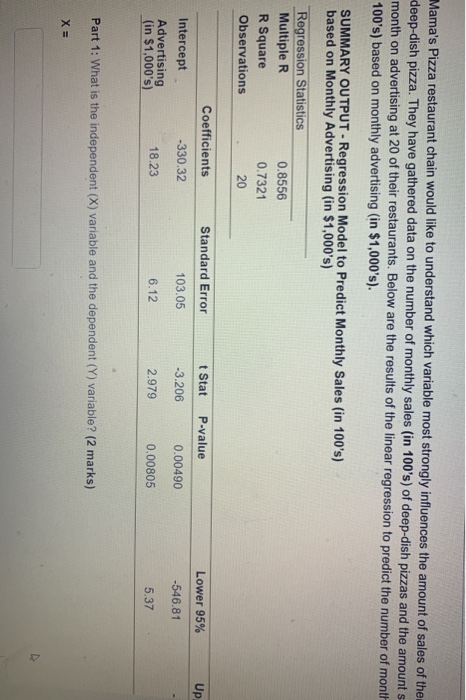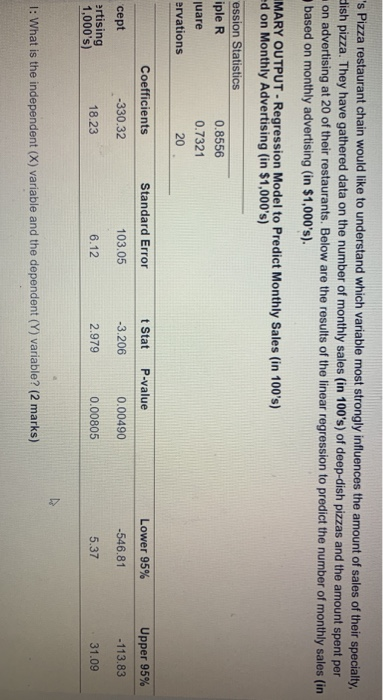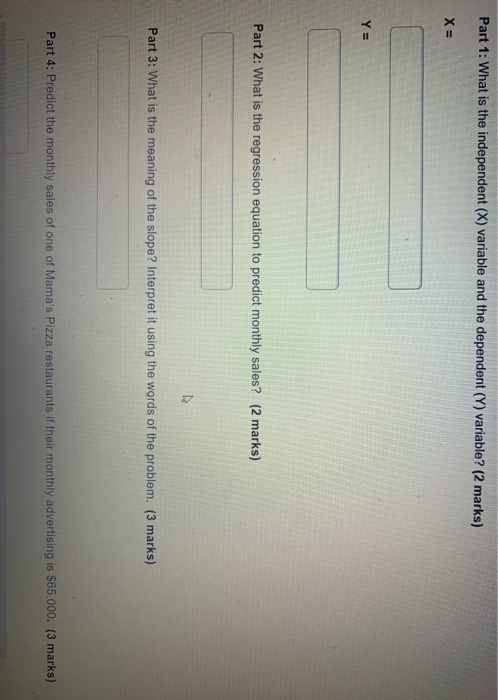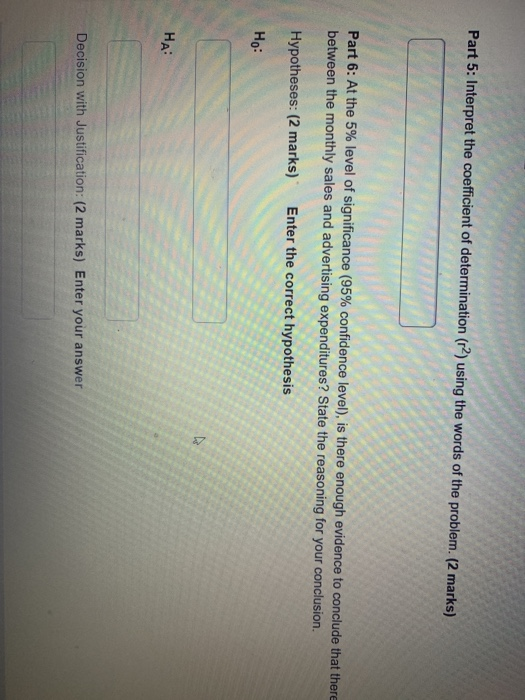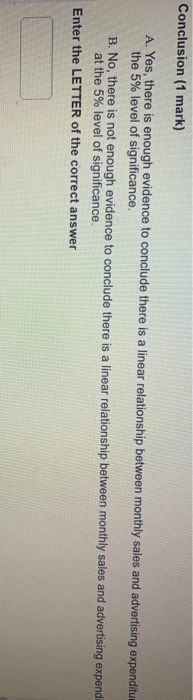Mama's Pizza restaurant chain would like to understand which variable most strongly influences the amount of sales of the deep-dish pizza. They have gathered data on the number of monthly sales (in 100's) of deep-dish pizzas and the amounts month on advertising at 20 of their restaurants. Below are the results of the linear regression to predict the number of month 100's) based on monthly advertising (in \$1,000's). SUMMARY OUTPUT - Regression Model to Predict Monthly Sales (in 100's) based on Monthly Advertising (in \$1,000's) Regression Statistics Multiple R 0.8556 R Square 0.7321 Observations 20 Coefficients Standard Error t Stat P-value Lower 95% Up -330.32 103.05 -3.206 0.00490 -546.81 Intercept Advertising (in \$1,000's) 18.23 6.12 2.979 0.00805 5.37 Part 1: What is the independent (X) variable and the dependent (Y) variable? (2 marks) X =
's Pizza restaurant chain would like to understand which variable most strongly influences the amount of sales of their specialty Llish pizza. They have gathered data on the number of monthly sales (in 100's) of deep-dish pizzas and the amount spent per on advertising at 20 of their restaurants. Below are the results of the linear regression to predict the number of monthly sales (in based on monthly advertising (in \$1,000's). MARY OUTPUT - Regression Model to Predict Monthly Sales (in 100's) ed on Monthly Advertising (in \$1,000's) ession Statistics iple R juare ervations 0.8556 0.7321 20 Coefficients Standard Error t Stat P-value Lower 95% Upper 95% -330.32 103.05 -3.206 0.00490 -546.81 - 113.83 cept artising 1,000's) 18.23 6.12 2.979 0.00805 5.37 31.09 1: What is the independent (X) variable and the dependent (Y) variable? (2 marks)
Part 1: What is the independent (X) variable and the dependent (Y) variable? (2 marks) X= Y= Part 2: What is the regression equation to predict monthly sales? (2 marks) Part 3: What is the meaning of the slope? Interpret it using the words of the problem. (3 marks) Part 4: Predict the monthly sales of one of Mama's Pizza restaurants if their monthly advertising is \$65,000. (3 marks)
Part 5: Interpret the coefficient of determination (2) using the words of the problem. (2 marks) Part 6: At the 5% level of significance (95% confidence level), is there enough evidence to conclude that there between the monthly sales and advertising expenditures? State the reasoning for your conclusion Hypotheses: (2 marks) Enter the correct hypothesis Ho: HA Decision with Justification: (2 marks) Enter your answer
Conclusion (1 mark) A. Yes, there is enough evidence to conclude there is a linear relationship between monthly sales and advertising expenditum the 5% level of significance. B. No, there is not enough evidence to conclude there is a linear relationship between monthly sales and advertising expendi at the 5% level of significance. Enter the LETTER of the correct answer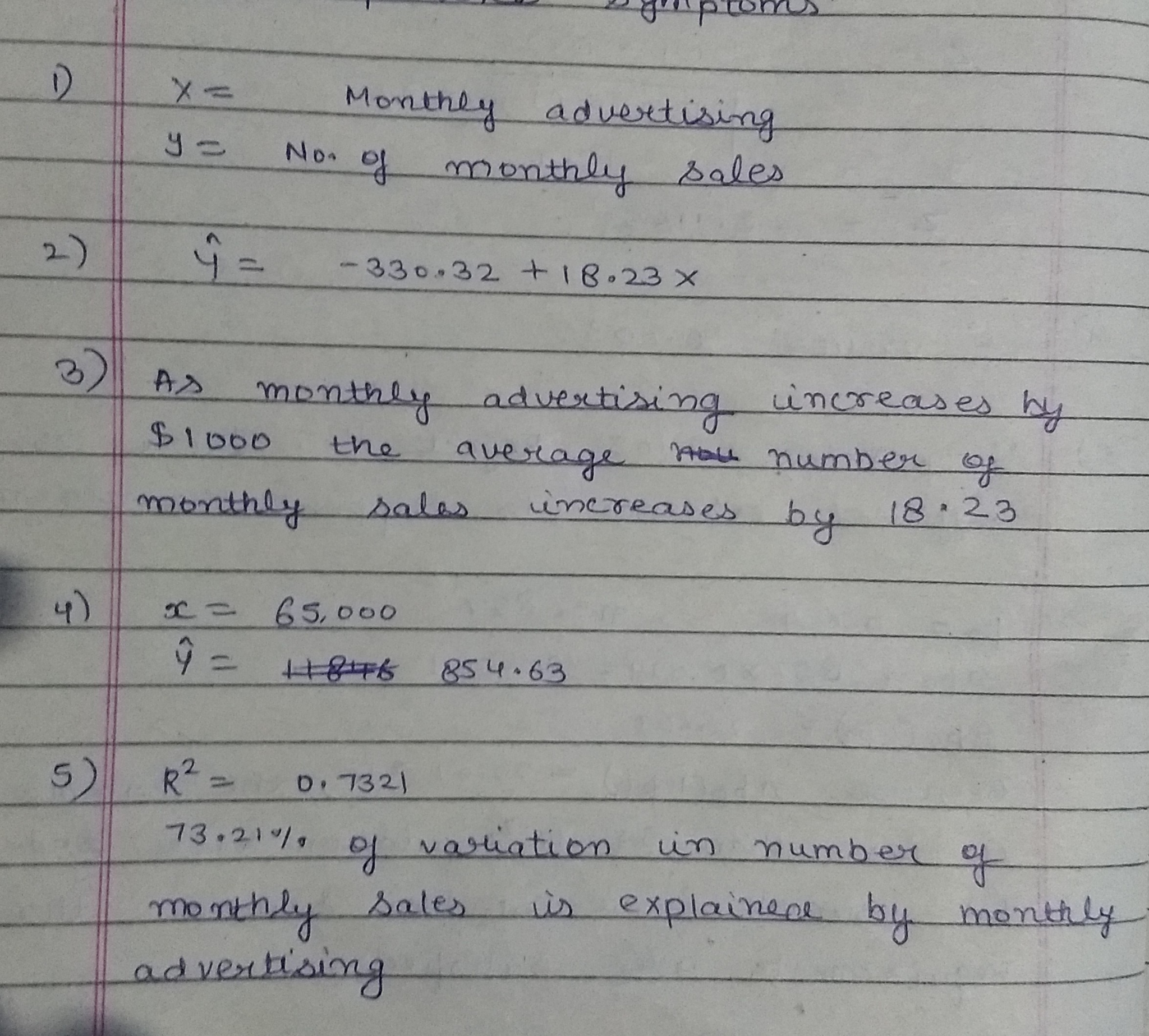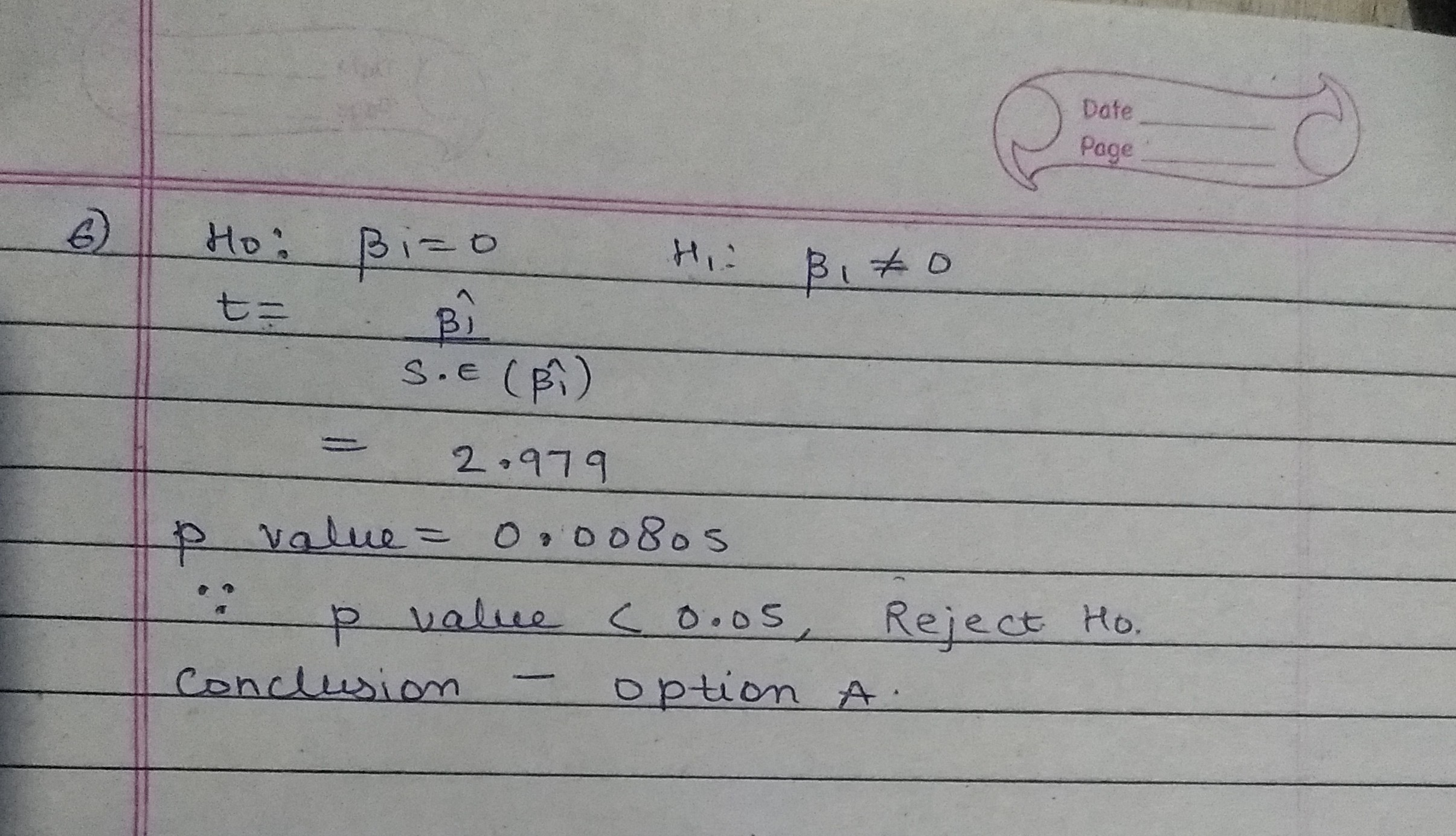##### Add Answer of: Mama's Pizza restaurant chain would like to understand which variable most strongly influences the amount of...
Similar Homework Help Questions
Free Homework App